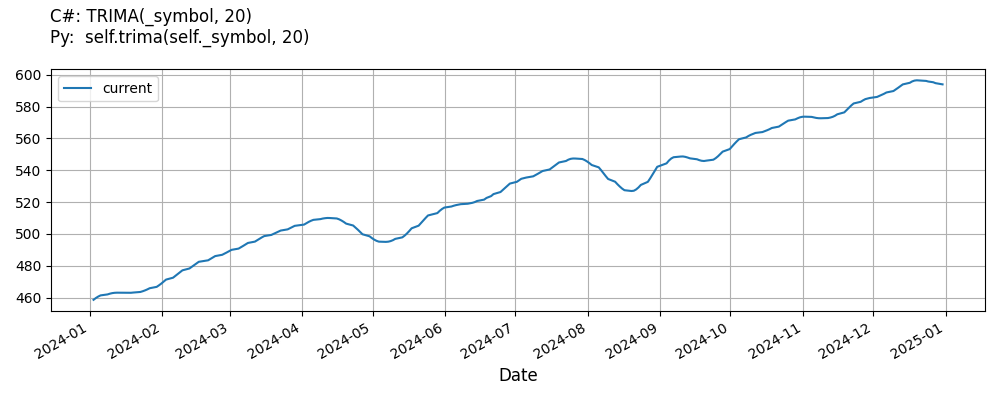# Supported Indicators

## Triangular Moving Average

### Introduction

This indicator computes the Triangular Moving Average (TRIMA). The Triangular Moving Average is calculated with the following formula: (1) When the period is even, TRIMA(x,period)=SMA(SMA(x,period/2),(period/2)+1) (2) When the period is odd, TRIMA(x,period)=SMA(SMA(x,(period+1)/2),(period+1)/2)

To view the implementation of this indicator, see the LEAN GitHub repository.

### Using TRIMA Indicator

To create an automatic indicators for TriangularMovingAverage, call the TRIMA helper method from the QCAlgorithm class. The TRIMA method creates a TriangularMovingAverage object, hooks it up for automatic updates, and returns it so you can used it in your algorithm. In most cases, you should call the helper method in the Initialize method.

public class TriangularMovingAverageAlgorithm : QCAlgorithm
{
private Symbol _symbol;
private TriangularMovingAverage _trima;

public override void Initialize()
{
_trima = TRIMA(_symbol, 20);
}

public override void OnData(Slice data)
{
{
// The current value of _trima is represented by itself (_trima)
// or _trima.Current.Value
Plot("TriangularMovingAverage", "trima", _trima);

}
}
}
class TriangularMovingAverageAlgorithm(QCAlgorithm):
def Initialize(self) -> None:
self.trima = self.TRIMA(self.symbol, 20)

def OnData(self, slice: Slice) -> None:
# The current value of self.trima is represented by self.trima.Current.Value
self.Plot("TriangularMovingAverage", "trima", self.trima.Current.Value)



The following reference table describes the TRIMA method:

### TRIMA()1/1

            TriangularMovingAverage QuantConnect.Algorithm.QCAlgorithm.TRIMA (
Symbol                           symbol,
Int32                            period,
*Nullable<Resolution>      resolution,
*Func<IBaseData, Decimal>  selector
)


Creates a new TriangularMovingAverage indicator.

If you don't provide a resolution, it defaults to the security resolution. If you provide a resolution, it must be greater than or equal to the resolution of the security. For instance, if you subscribe to hourly data for a security, you should update its indicator with data that spans 1 hour or longer.

You can manually create a TriangularMovingAverage indicator, so it doesn't automatically update. Manual indicators let you update their values with any data you choose.

Updating your indicator manually enables you to control when the indicator is updated and what data you use to update it. To manually update the indicator, call the Update method with time/number pair, or an IndicatorDataPoint. The indicator will only be ready after you prime it with enough data.

public class TriangularMovingAverageAlgorithm : QCAlgorithm
{
private Symbol _symbol;
private TriangularMovingAverage _trima;

public override void Initialize()
{
_trima = new TriangularMovingAverage(20);
}

public override void OnData(Slice data)
{
if (data.Bars.TryGeValue(_symbol, out var bar))
{
_trima.Update(bar.EndTime, bar.Close);
}

{
// The current value of _trima is represented by itself (_trima)
// or _trima.Current.Value
Plot("TriangularMovingAverage", "trima", _trima);

}
}
}
class TriangularMovingAverageAlgorithm(QCAlgorithm):
def Initialize(self) -> None:
self.trima = TriangularMovingAverage(20)

def OnData(self, slice: Slice) -> None:
bar = slice.Bars.get(self.symbol)
if bar:
self.trima.Update(bar.EndTime, bar.Close)

# The current value of self.trima is represented by self.trima.Current.Value
self.Plot("TriangularMovingAverage", "trima", self.trima.Current.Value)



To register a manual indicator for automatic updates with the security data, call the RegisterIndicator method.

public class TriangularMovingAverageAlgorithm : QCAlgorithm
{
private Symbol _symbol;
private TriangularMovingAverage _trima;

public override void Initialize()
{
_trima = new TriangularMovingAverage(20);
RegisterIndicator(_symbol, _trima, Resolution.Daily);
}

public override void OnData(Slice data)
{
{
// The current value of _trima is represented by itself (_trima)
// or _trima.Current.Value
Plot("TriangularMovingAverage", "trima", _trima);

}
}
}
class TriangularMovingAverageAlgorithm(QCAlgorithm):
def Initialize(self) -> None:
self.trima = TriangularMovingAverage(20)
self.RegisterIndicator(self.symbol, self.trima, Resolution.Daily)

def OnData(self, slice: Slice) -> None:
# The current value of self.trima is represented by self.trima.Current.Value
self.Plot("TriangularMovingAverage", "trima", self.trima.Current.Value)



The following reference table describes the TriangularMovingAverage constructor:

### TriangularMovingAverage()1/2

            TriangularMovingAverage QuantConnect.Indicators.TriangularMovingAverage (
string  name,
int     period
)


Initializes a new instance of the TriangularMovingAverage class using the specified name and period.

### TriangularMovingAverage()2/2

            TriangularMovingAverage QuantConnect.Indicators.TriangularMovingAverage (
int  period
)


Initializes a new instance of the TriangularMovingAverage class using the specified period.

### Visualization

The following image shows plot values of selected properties of TriangularMovingAverage using the plotly library.You can also see our Videos. You can also get in touch with us via Discord.